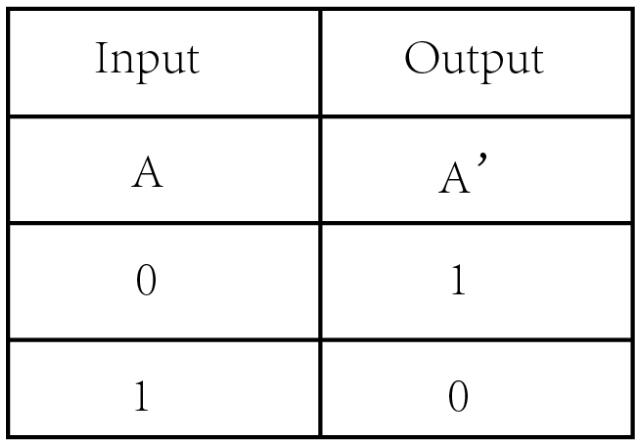# Crowbits-Logic NOT

Rating:
0% of 100
SKU
CRB0036S
Weight
15 g
Availability
In stock
\$3.20

What is the Crowbits-Logic NOT?

The logic NOT is a logic gate that uses one input signal to control one output signal, it creates rules for your circuit.

How does it work?

The interface of the logic NOT is a digital interface, it will output a logic-high or low (1 or 0) signal.

The logic NOT has one input and one output, the output does a reverse operation on the input, which means if you send a logic-high signal to the input, the logic NOT will output a logic-low signal.

Tips

The NOT gate, sometimes called a logic inverter, it reverse the logic state. If the input is “1”, then the output is “0”. If the input is 0, then the output is 1.Specification

Operating Voltage: 3.3V DC

Dimensions: 31.5(L)*24.5(W)*13(H) mm